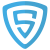# How to scrape data from HTML tables with Python Pandas

Did you know that Pandas can also do web scraping?

Pandas provides a method called `read_html` which supports reading tables from HTML content. We can pass the HTML content or the URL to a web page with tabular data directly. It is fairly straight forward in most cases, but there are cases where it’s a bit tricky to get it to work.

Web scraping with pandas is primarily useful for extracting basic HTML tables from a web page, if you just need a few pages. We don’t recommend web scraping with pandas, if you need to extract data that is not tabular, or if you need to scrape data from thousands of pages. Python packages such as LXML or beautifulsoup are better suited for that job.

Here is what we’ll try to do:

1. Load the first table with population data of countries from this URL https://scrapeme.live/population-data.html, into a Pandas Dataframe
2. Get the top 20 countries
3. Make the Rank column as the index.
4. Replace all NaN values with an empty string.
5. Extract the year from the Date column.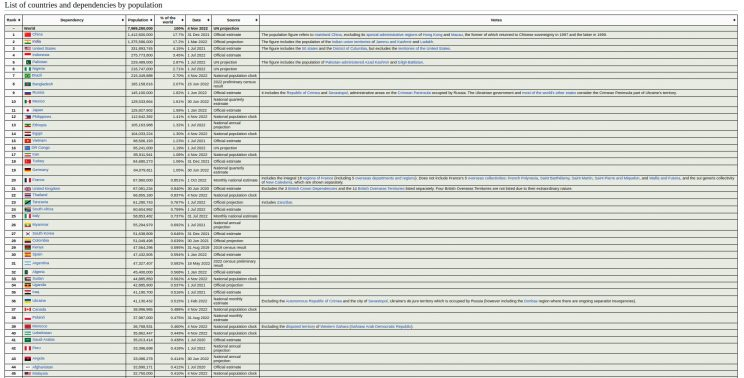Make sure you have these packages installed in Python

• Pandas
• LXML

Here is how to install them

`pip install pandas lxml`

## Load a specific table from a page with multiple tables using string or RegEx matching

Let’s import the required libraries and get started.

```# Import pandas
import pandas as pd```

Now, let’s read the table from the webpage using `read_html`

```# Fetch all the tables present in the HTML content
url = 'https://scrapeme.live/population-data.html'

If we check the length of the all_tables object, we can see that pandas has created two dataframes, one for the population data table, and the another for population statistics table below it.

```# Check the length of tables list
print(len(all_tables))```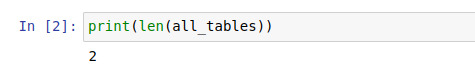We have multiple tables now. But how do we get only the required table from the webpage – ie. the first table?
In this example you can just use all_tables to get the first table. But that wouldn’t always be the case with other web pages that may have multiple HTML Tables.

```# Check the first table
all_tables```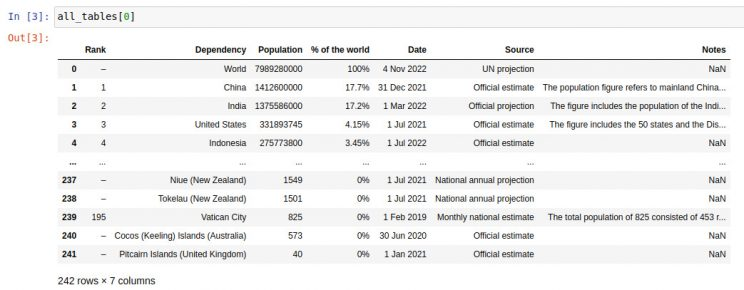```# Check the second table
all_tables```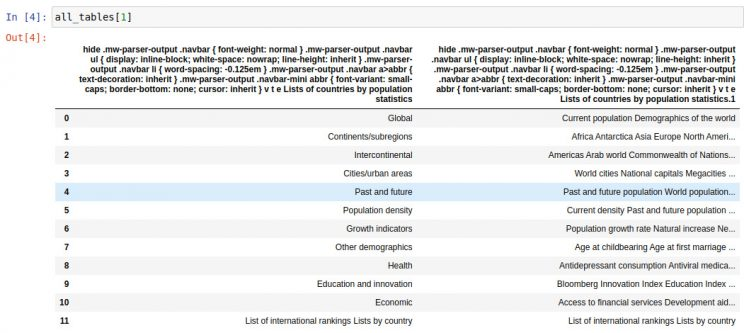read_html function has a parameter called match, which can be used to extract only those tables that matches a certain string or Regular Expression.

```# Fetching tables with the matching keyword

We will use the keyword “Rank” found on the table header to filter out all other tables. You just need to use a string or regex that is not common to other tables in the HTML page.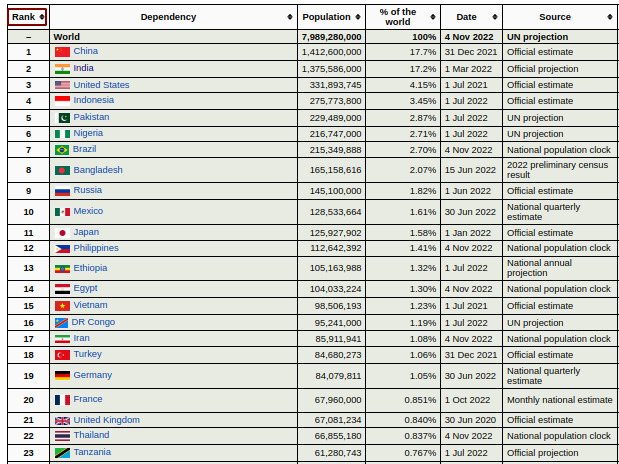```# Check the length of table
print(len(all_tables))```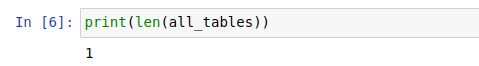There we have it, just 1 table.

## Limit the number of rows extracted

We have 241 rows. For this example, we will limit the data to the first 20 rows. We could just use the head method of the resulting data frame. But let’s try another way.
We can use the parameter skiprows, which takes an integer or a list as arguments skipping any number of rows from the extracted table.
```# fetch the required table with the first 20 rows
```

## Set the index of the dataframe directly in read_html

Let’s try and set the set Rank as the index of the data frame. You can always do this later by using the set_index method of a dataframe. Interestingly, read_html has a parameter called `index_col` which takes in the name of the index column, and sets it as index.

```# Setting Rank column as index column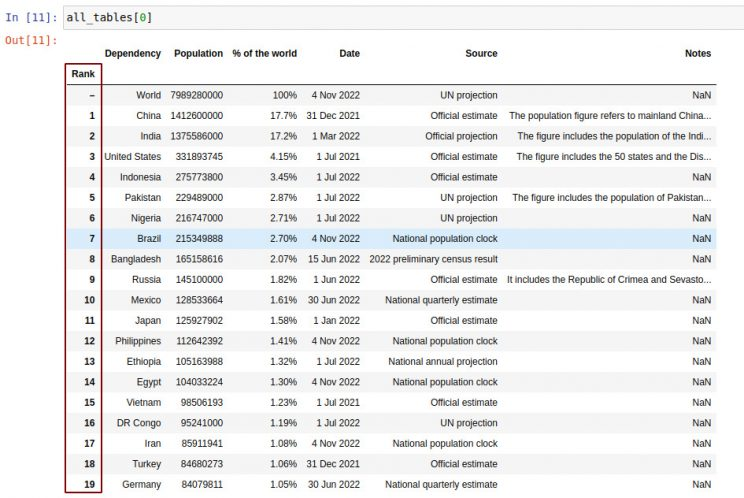## Transform a column using converter functions

The column date is in the form 4 Nov 2022 . Let’s just get the year. To convert the Date column, we can use the parameter converter. It takes a dictionary as input, where key is the column name that needs to be transformed and value is a function that takes a single argument, which is the cell content.

```# Transforming the Date column
from datetime import datetime

def get_year(data_string):
return datetime.strptime(data_string, '%d %b %Y').year

all_tables = pd.read_html(url,match='Rank',skiprows=list(range(21,243)),index_col = 'Rank',converters = {'Date': get_year })```## Parsing dates

We can also use the parameter `parse_dates` to change the date format.

```# Make column Date into Date data type

## Replacing NaN with empty string

You can see some `NaN` values in the dataset, which represent empty values. We can remove the `NaN` values with custom values by using the parameter `keep_default_na`. You can find an example below:

```# Replace NaN fields
all_tables = pd.read_html(url,match='Rank',skiprows=list(range(21,243)),index_col = 'Rank',converters = {'Date': get_year },keep_default_na=False)

```

## Exporting to CSV

Lets write this data into a CSV file which can be opened in Excel.
Pandas supports exporting data into multiple formats such as JSON, CSV, Excel, and HTML.
Here, we will write the data into a CSV file population_data.csv.

```# getting the required table from list
population_table = all_tables
# saving CSV in the current working directory
population_table.to_csv('population_data.csv', index=False)```

We can exclude the index column(Rank) from the CSV by setting the parameter `index` to `False`.

Let’s try something a little bit harder, to show you some more features of read_html in pandas.

This time, let’s scrape a table with product comparison data from Amazon.com – https://www.amazon.com/Apple-iPhone-Fully-Unlocked-128/dp/B07P611Q4N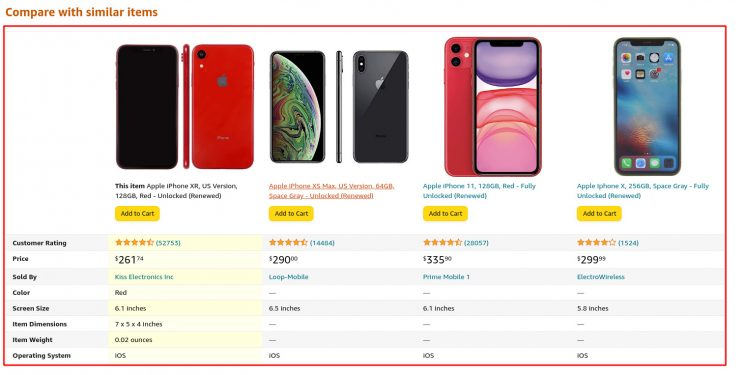Let’s first try to fetch the table from the URL using the `read_html()` method.

```# Fetch all the tables present in the HTML content
url = 'https://www.amazon.com/Apple-iPhone-Fully-Unlocked-128/dp/B07P611Q4N'

Instead of giving us a list of table, we have a HTTP 503 Error, as shown below.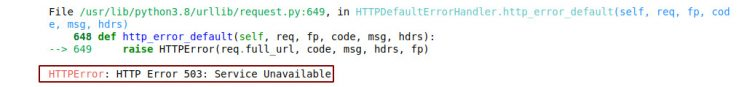We can see that the error is returned from the `urllib` module. The `read_html()` method uses `urllib` to send requests. This 503 error happens because Amazon.com knows this request is not coming from a browser, as it doesn’t have any of the needed HTTP headers.

This may also occur due to the website blocking access using anti scraping measures which may not be as simple to fix.

Luckily for this problem we have a simple fix of telling Amazon that you are using a browser by passing certain browser specific HTTP headers.

But, Pandas does not let us change the HTTP headers.

As a workaround, we will use the python requests library to first download the HTML with the right set of HTTP headers and then give just that downloaded HTML content of the page to pandas to parse and read the tables.

To install requests:

```pip install requests
```
```# HTTP request to amazon using python requests module
import requests

'authority': 'www.amazon.com',
'accept': 'text/html,application/xhtml+xml,application/xml;q=0.9,image/avif,image/webp,image/apng,*/*;q=0.8,application/signed-exchange;v=b3;q=0.9',
'accept-language': 'en-GB,en-US;q=0.9,en;q=0.8',
'cache-control': 'no-cache',
'device-memory': '8',
'dpr': '1',
'ect': '4g',
'pragma': 'no-cache',
'rtt': '100',
'sec-ch-device-memory': '8',
'sec-ch-dpr': '1',
'sec-ch-ua-mobile': '?0',
'sec-ch-ua-platform': '"Linux"',
'sec-ch-viewport-width': '1920',
'sec-fetch-dest': 'document',
'sec-fetch-mode': 'navigate',
'sec-fetch-site': 'none',
'sec-fetch-user': '?1',
'user-agent': 'Mozilla/5.0 (X11; Linux x86_64) AppleWebKit/537.36 (KHTML, like Gecko) Chrome/106.0.0.0 Safari/537.36',
'viewport-width': '1920',
}

# checking the status code and obtaining the html response content
if(response.status_code == 200):
html_content = response.text```

Now, pass the HTML content to Pandas `read_html()` and extract the required table.

```# Fetching html content using python requests
html_content = get_response_text(url)

# fetching all the tables in html content passed

## Load a specific table from a page with multiple tables using HTML attributes

Check the number of tables extracted

```# Check the length of tables list
print(len(all_tables))```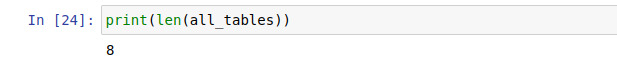We can observe that many unwanted tables are being fetched. To get the required table, we can use the parameter `attrs`. This parameter takes a dictionary of HTML attributes that can be used to identify the table from the HTML content.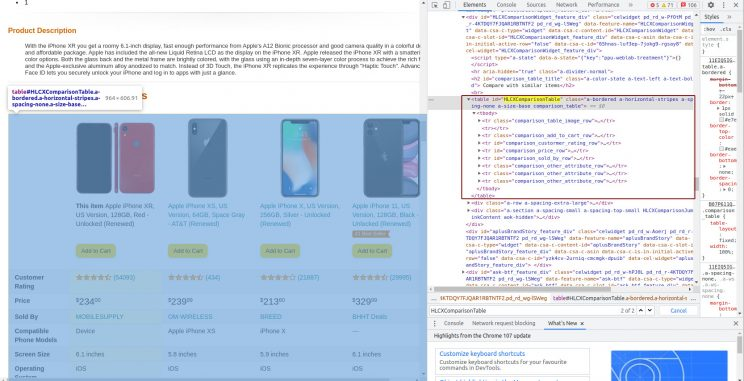From the above screenshot, we can see that the required table has an attribute id which is `HLCXComparisonTable`. This can be used to identify the table in the HTML content.

```# fetching the required table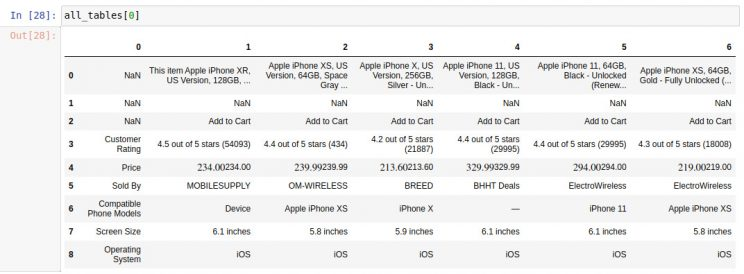If the attributes(`attrs`) are not matching with any table in the HTML content, Pandas will raise a `Value Error` saying no table found. An example is given below:

```# fetching the required table

Here, table `id` should match `table_name_not_to_be_found` which is not present in the webpage.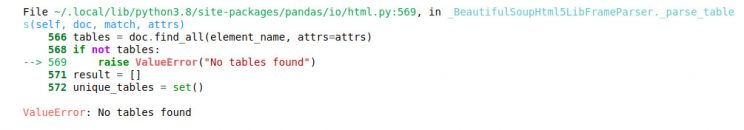## Exporting to JSON

Finally, the data is ready. Let’s write it into a JSON file –  Comparison_table.json.

```# Getting the required table from list
comparison_tables = all_tables

# saving JSON in the current working directory
comparison_tables.to_json('Comparison_table.json', index=False)```

Thats it! If you have any questions or run into any problems, feel free to write a comment below.

Posted in:   Web Scraping Tutorials

## Turn the Internet into meaningful, structured and usable data